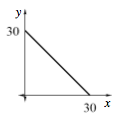### Home > INT3 > Chapter Ch1 > Lesson 1.1.3 > Problem1-39

1-39.

Jill needs to cut a smaller piece from a $30$-foot length of lumber. Create multiple representations (table, graph, and equation) for the function with $x$‑values that are the length of the piece Jill cuts off and $y$-values that are the length of the piece that is left over. Which representation best portrays the situation? Why? Explain.

Make a table.

What input and output values represent the length of the lumber before she cuts it?

 $x$ $0$ $1$ $6$ $20$ $y$ $30$ $29$ $24$ $10$

Draw a graph. What is the slope?What is the $y$-intercept? What is the equation?

$y = 30 - x$

Decide which representation best shows that when she cuts off a longer piece, the remaining piece is shorter,